# New Pattern Quantitative Aptitude Questions Based on IBPS PO Mains 2018 (Day-02)

Dear Aspirants, Quantitative Aptitude plays a crucial role in Banking and all other competitive exams. To enrich your preparation, here we have provided New Pattern Aptitude Questions that are similar to the questions asked in IBPS PO Mains 2018. Candidates those who are going to appear in upcoming bank exams like IBPS Clerk, IBPS SO, etc., can practice these questions daily and make your preparation effective.

[WpProQuiz 4513]

Direction (1 – 5): Read the following information carefully and answer the given questions:

Total distance between A to B is 240km. Train P starts running with an average speed of (S) kmph from A to B while another train Q starts running after 2 hours of train A and reaches B 60 minutes before train P. If train A stops for 60 minutes at a line crossing and second train did not stop at any place, then the ratio between the speed of train P to speed of train Q is 4:5.

Prem distributed Rs.14000 between his two daughters Anu and Bharathi and both of them invested at the rate of (S-6)% SI per annum. The age of Bharathi and Anu at that time was 18 years and (S) years respectively and such that each daughter may get equal amounts, when they attain the age of 21 years. Initial amount the Anu has is (T).

The price of article increased by (S)% every year. If the difference between the price at the end of the third year and fourth years is (U) and the price at the end of second year is Rs.23200.

Prem sells his car, if profit on selling a car for (T) is thrice the loss on selling it for Rs.4500. The Cost price of Prem car is (V).

1) Find the value in the place of (S)

a) 15 kmph

b) 26 kmph

c) 16 kmph

d) 18 kmph

e) None of these

2) Find the value in the place of (V)

a) Rs.8000

b) Rs.6000

c) Rs.4000

d) Rs.5000

e) None of these

3) Quantity I: U

Quantity II: T

a) Quantity I > Quantity II

b) Quantity I ≥ Quantity II

c) Quantity II > Quantity I

d) Quantity II ≥ Quantity I

e) Quantity I = Quantity II or Relation cannot be established

4) Find the difference between V and T?

a) Rs.1500

b) Rs.2500

c) Rs.500

d) Rs.1600

e) None of these

5) Find the value in the place of (U)

a) 4305.92

b) 4206.92

c) 4108.92

d) 4006.92

e) None of these

Directions (6 – 10): The questions below are based on the given Series-I. The series-I satisfy a certain pattern, follow the same pattern in Series-II and answer the questions given below.

6)

I. 3256, 3260, 3251, 3276, 3227, 3348

II. 1089 …… 1301. If 1301 is the nth term, then find the value of n?

a) 5

b) 6

c) 7

d) 9

e) 8

7)

I. 184, 356, 969, 2207, 4286, 7454

II. 42, 211 …. 4132. If 4132 is the nth term, what value should come in the place of (n+1)th term?

a) 2207

b) 8117

c) 9207

d) 7297

e) 7587

8)

I. 179, 195, 212, 248, 359, 807

II. 192, 211…… 922. If 922 is the nth term, then find the value of n?

a) 11

b) 10

c) 9

d) 7

e) 6

9)

I. 64, 33, 34, 52, 105, 263.5

II. 72 ….. 881.5. If 881.5 is the nth term, then what value should come in the place of (n-2)th term?

a) 117

b) 38

c) 58

d) 293.5

e) 457.5

10)

I. 722, 850, 994, 1202, 1538, 2386

II. 84, 256, 446 ….. 4230. If 4230 is the nth term, find the value of (n+1)?

a) 11

b) 10

c) 9

d) 8

e) 7

Direction (1 – 5):

Let us take the original time taken to cross 240 km distance be x hours

Time taken by train P to reach B =(X+1)

Time taken by train Q to reach B=(x+1)-2-1=(x-2)

Speed of first train=240/(x+1)

Speed of second train=240/(x-2)

(240/(x+1))/(240/(x-2))=4/5

(x-2)/(x+1)=4/5

5x-10=4x+4

X=14

Time taken by train P =15 hrs

Time taken by train Q =12 hrs

Speed of first train (S) =240/15=16 kmph

Let the amount received by Anu=A

Let the amount received by Bharathi=14000-A

A+(A*5*10/100)=(14000-A)+((14000-A)*10*3/100)

A+A/2=(14000-A)+4200-3A/10

3A/2=18200-A-3A/10

3A/2+A+3A/10=18200

28A=182000

A=6500

Initial amount=a

Second years=a*116/100

116a/100=23200

a=20000

Third year=a*(116/100)*(116/100)

Fourth year=a*(116/100)*(116/100)*(116/100)

Difference of fourth and third year (U)=a*(116/100)*(116/100)*(116/100-1)

U=20000*(116/100)*(116/100)*(16/100)

Difference of fourth and third year (U) = 4305.92

Cost price of car=V

6500-V=3(V-4500)

6500-V=3V-13500

4V=20000

V=5000

Speed of first train (S) =240/15=16 kmph

V=5000

Quantity I:

Difference of fourth and third year (U) = 4305.92

Quantity II:

Quantity I < Quantity II

V=5000

Required difference = 6500 – 5000 = Rs.1500

Difference of fourth and third year (U) = 4305.92

Directions (6 – 10):

Series –I pattern:

3256 is the 1st term

3256 + 22 = 3260

3260 – 32 = 3251

3251 + 52 = 3276

3276 – 72 = 3227

3227 + 112 = 3348 = 6th term

Series –II pattern:

1089 is the 1st term

1089 + 22 = 1093

1093 – 32 = 1084

1084 + 52 = 1109

1109 – 72 = 1060

1060 + 112 = 1181

1181 – 132 = 1012

1012 + 172 = 1301 = 8th term

Series – I pattern: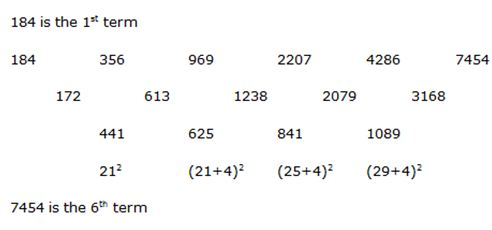Series –II pattern: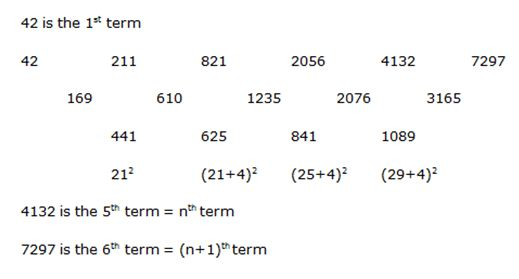Series –I pattern: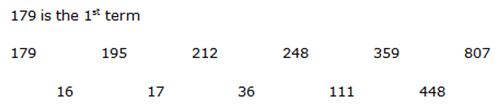Pattern of the difference in the above series is:

16 * 1 + 1 = 17

17 * 2 +2 = 36

36 * 3 + 3 = 111

111 * 4+ 4 = 448

807 is 6th term

Series – II pattern: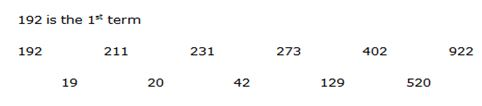Pattern of the difference in the above series is:

19 * 1 + 1 = 20

20 * 2 + 2 = 42

42 * 3+ 3 = 129

129 * 4 + 4 = 520

922 is 6th term

Series –I pattern:

64 is the 1st term

64 * 0.5 + 1 = 33

33 * 1 + 1 = 34

34 * 1.5 + 1 = 52

52 * 2 + 1 = 105

105 * 2.5 + 1 = 263.5 = 6th term

Series –II pattern:

72 is the 1st term

72 * 0.5 + 1 = 37

37 * 1 + 1 = 38

38 * 1.5 + 1 = 58

58 * 2 + 1 = 117 = 5th term = (n-2)th term

117 * 2.5 + 1 = 293.5 = 6th term = (n-1)th term

293.5 * 3 + 1 = 881.5 = 7th term = nth term

Series – I pattern: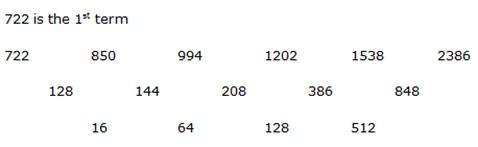Pattern of the difference of difference in the above series is:

16 * 4 = 64

64 * 2 = 128

128 * 4 = 512

2386 is the 6th term

Series –II pattern: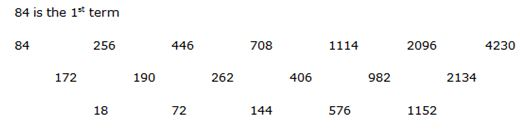Pattern of the difference of difference in the above series is:

18 * 4 = 72

72 * 2 = 144

144 * 4 = 576

576 * 2 = 1152

4230 is the 7th term = nth term

(n +1) = 7 +1 = 8

5 1 vote
Rating